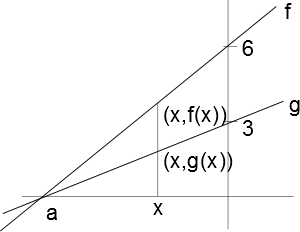Suppose you have two linear functions $f$ and $g$ as shown in the figure. Then $\displaystyle \lim_{x\to a} \frac{f(x)}{g(x)}$ A. is equal to 2. B. is equal to 3. C. does not exist. D. cannot be determined because there is not enough information. In the answer box below, explain your reasoning for the choice you made above. Use complete sentences and correct grammar, spelling, and punctuation. Be specific and detailed. Write as if you were explaining the answer to someone else in class.In order to get credit for this problem all answers must be correct.